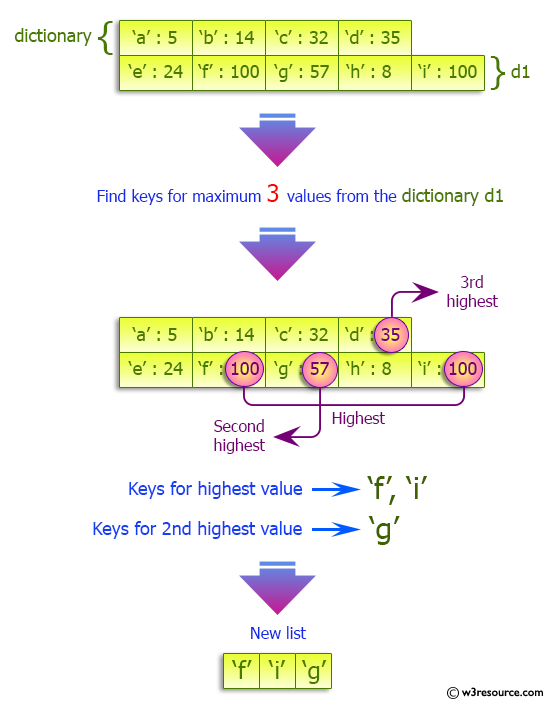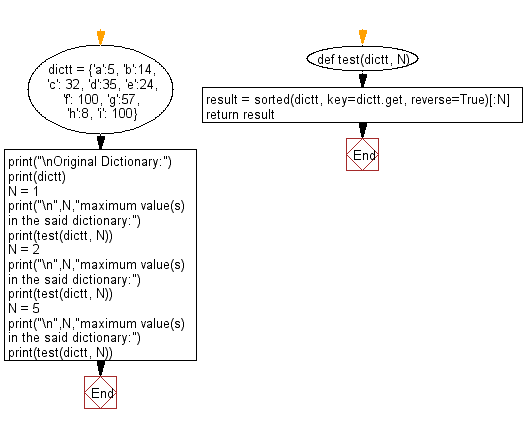﻿ Python: Find the specified number of maximum values in a given dictionary - w3resource# Python: Find the specified number of maximum values in a given dictionary

## Python dictionary: Exercise-59 with Solution

Write a Python program to find the specified number of maximum values in a given dictionary.

Sample Solution:

Python Code:

``````def test(dictt, N):
result = sorted(dictt, key=dictt.get, reverse=True)[:N]
return result
dictt = {'a':5, 'b':14, 'c': 32, 'd':35, 'e':24, 'f': 100, 'g':57, 'h':8, 'i': 100}
print("\nOriginal Dictionary:")
print(dictt)
N = 1
print("\n",N,"maximum value(s) in the said dictionary:")
print(test(dictt, N))
N = 2
print("\n",N,"maximum value(s) in the said dictionary:")
print(test(dictt, N))
N = 5
print("\n",N,"maximum value(s) in the said dictionary:")
print(test(dictt, N))
```
```

Sample Output:

```Original Dictionary:
{'a': 5, 'b': 14, 'c': 32, 'd': 35, 'e': 24, 'f': 100, 'g': 57, 'h': 8, 'i': 100}

1 maximum value(s) in the said dictionary:
['f']

2 maximum value(s) in the said dictionary:
['f', 'i']

5 maximum value(s) in the said dictionary:
['f', 'i', 'g', 'd', 'c']
```

Pictorial Presentation:Flowchart:## Visualize Python code execution:

The following tool visualize what the computer is doing step-by-step as it executes the said program:

Python Code Editor:

Have another way to solve this solution? Contribute your code (and comments) through Disqus.

What is the difficulty level of this exercise?

Test your Programming skills with w3resource's quiz.

﻿

## Python: Tips of the Day

Invokes the provided function after ms milliseconds:

Example:

```from time import sleep

def tips_delay(fn, ms, *args):
sleep(ms / 1000)
return fn(*args)
print(tips_delay(
lambda x: print(x),
1000,
'w3r'
))
```

Output:

```w3r
None
```